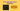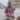# 5 JavaScript Scope Gotchas

Posted April 26, 2020Start discussion

In JavaScript, a code block, a function, or module create scopes for variables. For example, the `if` code block creates a scope for the variable `message`:

``````if (true) {
const message = 'Hello';
console.log(message); // 'Hello'}
console.log(message); // throws ReferenceError``````

`message` is accessible inside the scope of `if` code block. However, outside of the scope, the variable is not accessible.

Ok, that was a short intro to scopes. If you’d like to learn more, I recommend reading my post JavaScript Scope Explained in Simple Words .

What follows are 5 interesting cases when the JavaScript scope behaves differently than you expect. You might study these cases to improve your knowledge of scopes, or just to prepare for a fancy coding interview.

## 1. var variables inside for cycle

Consider the following code snippet:

``````const colors = ['red', 'blue', 'white'];

for (let i = 0, var l = colors.length; i < l; i++) {
console.log(colors[i]); // 'red', 'blue', 'white'
}
console.log(l); // ???
console.log(i); // ???``````

What happens when you log `l` and `i` variables?

`console.log(l)` logs the number `3`, while `console.log(i)` throws a `ReferenceError`.

The `l` variable is declared using a `var` statement. As you might know already, `var` variables are scoped only by a function body and not by a code block.

On the opposite, the variable `i` is declared using a `let` statement. Because `let` variables are block scoped, `i` is accessible only within the scope of `for` cycle.

### The fix

Change `l` declaration from `var l = colors.length` to `const l = colors.length`. Now the variable `l` is encapsuled inside `for` cycle body.

## 2. function declaration inside code blocks

In the following code snipped:

``````// ES2015 env
{
function hello() {
return 'Hello!';
}
}

hello(); // ???``````

What happens when you invoke `hello()`? (consider the snippet is executed in ES2015 environment)

Because the code block creates a scope for the function declaration, invoking `hello()` (in ES2015 environment) throws `ReferenceError: hello is not defined`.

Interestingly that in a pre-ES2015 environment executing the above code snippet works without throwing errors. Do you know why? If so, please write your answer in a comment below!

## 3. Where can you import a module?

Can you import a module inside a code block?

``````if (true) {
import { myFunc } from 'myModule'; // ???
myFunc();
}``````

The script above would trigger an error: `'import' and 'export' may only appear at the top-level`.

You can import a module only at the topmost scope of the module file, also named the module scope.

### The fix

Always import modules from the module scope. Plus a good practice is to place the `import` statements at the beginning of the source file:

``````import { myFunc } from 'myModule';

if (true) {
myFunc();
}``````

ES2015 modules system is static. The modules dependencies are determined by analyzing the JavaScript source code, without executing it. Thus you cannot have `import` statements inside code blocks or functions since they are executed during runtime.

## 4. Function parameters scope

Consider the following function:

``````let p = 1;

function myFunc(p = p + 1) {
return p;
}

myFunc(); // ???``````

What happens when `myFunc()` is invoked?

When the function is invoked `myFunc()`, an error is thrown: `ReferenceError: Cannot access 'p' before initialization`.

It happens because the function parameters have their own scope (separated from the function scope). The parameter `p = p + 1` is equivalent to `let p = p + 1`.

Let’s take a closer look at `p = p + 1`.

First, a variable `p` is defined. Then JavaScript tries to evaluate the default value expression `p + 1`, but the binding `p` is already created but not yet initialized (the variable `let p = 1` of the outer scope is not accessed). Thus an error is thrown that `p` is accessed before initialization.

### The fix

To fix the problem, you can either rename the variable `let p = 1`, or rename the function parameter `p = p + 1`.

Let’s choose to rename the function parameter:

``````let p = 1;

function myFunc(q = p + 1) {
return q;
}

myFunc(); // => 2``````

The function parameter was renamed from `p` to `q`. When the invocation happens `myFunc()`, the argument is not specified, thus the parameter `q` is initialized with a default value `p + 1`. To evaluate `p + 1`, the variable `p` of the outer scope is accessed: `p + 1 = 1 + 1 = 2`.

## 5. Function declaration vs class declaration

The following code defines a function and a class inside of a code block:

``````if (true) {
function greet() {
// function body
}

class Greeter {
// class body
}
}

greet();       // ???
new Greeter(); // ???``````

Are both `greet` and `Greeter` accessible outside of the block scope? (consider ES2015 environment)

Both `function` and `class` declarations are block scoped. So invoking the function `greet()` and constructor `new Greeter()` outside of if code block scope throw a `ReferenceError`.

## 6. Summary

Care must be taken with `var` variables because they are function scoped, even being defined inside a code block.

Because the ES2015 modules system is static, you have to use the `import` syntax (as well as `export`) at the module scope.

The function parameters have their scope. When setting up a default parameter value, be sure that the variables inside the default expression are initialized with values.

In an ES2015 runtime environment, the function and class declarations are block scoped. However, a pre-ES2015 env, the function declarations are only function scoped.

Hopefully, these gotchas have helped you solidify your scope knowledge!

What other scope gotchas have you encountered?

## Quality posts into your inbox

I regularly publish posts containing:

• Important JavaScript concepts explained in simple words
• Overview of new JavaScript features
• How to use TypeScript and typing
• Software design and good coding practices